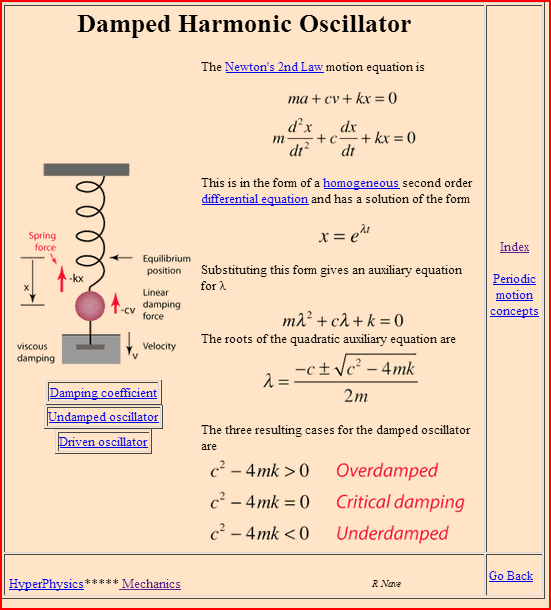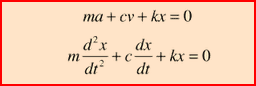# In what cases does dimensional analysis fail?

• I
Summary:
In what cases does dimensional analysis fails? And also is there like any preferred situation or an 'ideal' situation in order to use dimensional analysis? Thanks in advance!
It possible a diagram would be really really helpful

## Answers and Replies

berkeman
Mentor
Is this a schoolwork question?

No no Like they were just going through dimensional analysis in our class and I just thought well obviously this won't work for evert case so I just wanted to know what are those cases.

berkeman
Mentor
Summary: In what cases does dimensional analysis fails?
Well, a number of quantities are dimensionless, but I'm not sure that represents a failure. Can you think of some dimensionless constants or quantities that are used commonly?

Also, these two PF Insights articles would probably be good background reading for you to help understand dimensional analysis better...https://www.physicsforums.com/insights/learn-the-basics-of-dimensional-analysis/
https://www.physicsforums.com/insights/make-units-work/

•VVS2000
Vanadium 50
Staff Emeritus
Science Advisor
Education Advisor
I just thought well obviously this won't work for evert case so I just wanted to know what are those cases.

That's like asking when addition doesn't work - when it's the wrong tool for the job. Maybe you need to subtract or to multiply.

Dimensional analysis cannot tell you if the circumference of a circle is 6r, 2πr or 7r. It can tell you it is not 1/r or r2.

•anorlunda
Well, a number of quantities are dimensionless, but I'm not sure that represents a failure. Can you think of some dimensionless constants or quantities that are used commonly?

Also, these two PF Insights articles would probably be good background reading for you to help understand dimensional analysis better...https://www.physicsforums.com/insights/learn-the-basics-of-dimensional-analysis/
https://www.physicsforums.com/insights/make-units-work/
Thanks for the links!

•berkeman
That's like asking when addition doesn't work - when it's the wrong tool for the job. Maybe you need to subtract or to multiply.

Dimensional analysis cannot tell you if the circumference of a circle is 6r, 2πr or 7r. It can tell you it is not 1/r or r2.
No no not like that. What if sometimes we don't know the nature of the opposing quantity. How are you going to proceed then?

Vanadium 50
Staff Emeritus
Science Advisor
Education Advisor
What if sometimes we don't know the nature of the opposing quantity.

I don't understand.

I don't understand.
Like in damping of harmonic oscillations.

berkeman
Mentor
Like in damping of harmonic oscillations.
What do the overall dimensions of the quantity in the exponent need to be? So what does that tell you about the dimensions of λ ?

http://hyperphysics.phy-astr.gsu.edu/hbase/oscda.htmlVanadium 50
Staff Emeritus
Science Advisor
Education Advisor
I still don't understand. Perhaps you could use complete sentences? Maybe even paragraphs?

•nasu and SammyS
I still don't understand. Perhaps you could use complete sentences? Maybe even paragraphs?
For example damping in oscillations. What if we dont know the nature of damping? How do you find the equations of motion?

Vanadium 50
Staff Emeritus
Science Advisor
Education Advisor
How do you find the equations of motion?

What does that have to do with dimensional analysis?

berkeman
Mentor
For example damping in oscillations. What if we dont know the nature of damping? How do you find the equations of motion?
Please see post #10.

What does that have to do with dimensional analysis?
I am asking using dimensional analysis how do you find the eqaution of motion then

Please see post #10.
Sorry I am new here. Post 10 where?

berkeman
Mentor
No worries. The post number in each thread is shown in the upper right of each post. This post of mine will likely be #17, since I'm replying to your post #16. So just scroll up the thread to look for my reply in post #10. It was a post showing some equations from Hyperphysics to help you see the typical differential equation and solution for a damped harmonic oscillator.No worries. The post number in each thread is shown in the upper right of each post. This post of mine will likely be #17, since I'm replying to your post #16. So just scroll up the thread to look for my reply in post #10. It was a post showing some equations from Hyperphysics to help you see the typical differential equation and solution for a damped harmonic oscillator.That's actually really helpful. Thanks.

That's actually really helpful. Thanks.
So I cannot use dimensional analysis in such cases?

No worries. The post number in each thread is shown in the upper right of each post. This post of mine will likely be #17, since I'm replying to your post #16. So just scroll up the thread to look for my reply in post #10. It was a post showing some equations from Hyperphysics to help you see the typical differential equation and solution for a damped harmonic oscillator.So I cannot use dimensional analysis in such cases?

berkeman
Mentor
So I cannot use dimensional analysis in such cases?
Like in the Hyperphysics equations? Sure. Can you show the dimensions for each of the terms in the initial differential equation? And then show how that helps to define the dimensions of λ in the end?

Like in the Hyperphysics equations? Sure. Can you show the dimensions for each of the terms in the initial differential equation? And then show how that helps to define the dimensions of λ in the end?
I think I can. I can show the complimentary function for x. Then I can By eliminating the constants by plugging in the initial conditions, we can find the dimensions of lambda

berkeman
Mentor
I think I can. I can show the complimentary function for x. Then I can By eliminating the constants by plugging in the initial conditions, we can find the dimensions of lambda
Okay, but what are the dimensions (or units) for each of the terms in these equations?Chestermiller
Mentor
I think I can. I can show the complimentary function for x. Then I can By eliminating the constants by plugging in the initial conditions, we can find the dimensions of lambda
The dimensions of c are...?
The dimensions of m are...?
The dimensions of ##\lambda## are...?

The dimensiona of lambda is (time)^-1, time inverse, basically frequency. .M is mass. That is given. I dont or I am not quite sure on c